# Examples of FFTrees

## Examples of FFTs with FFTrees

This vignette illustrates how to construct fast-and-frugal trees (FFTs) for additional datasets included in the FFTrees package. (See Phillips et al., 2017, for a comparison across 10 real-world datasets.)

### Mushrooms dataThe mushrooms dataset contains data about mushrooms (see ?mushrooms for details). The goal of our model is to predict which mushrooms are poisonous based on 22 cues ranging from the mushroom’s odor, color, etc.

Here are the first few rows and a subset of 10 potential predictors of the mushrooms data:

Table 1: Binary criterion variable poisonous and 10 potential predictors in the mushrooms data.
poisonous cshape csurface ccolor bruises odor vcolor ringnum ringtype sporepc population habitat
TRUE x s n TRUE p w o p k s u
FALSE x s y TRUE a w o p n n g
FALSE b s w TRUE l w o p n n m
TRUE x y w TRUE p w o p k s u
FALSE x s g FALSE n w o e n a g
FALSE x y y TRUE a w o p k n g

#### Creating FFTs

Let’s create some trees using FFTrees()! We’ll use the train.p = .50 argument to split the original data into a $$50$$% training set and a $$50$$% testing set:

# Create FFTs from the mushrooms data:
set.seed(1) # for replicability of the training / test data split

mushrooms_fft <- FFTrees(formula = poisonous ~.,
data = mushrooms,
train.p = .50,   # split data into 50:50 training/test subsets
main = "Mushrooms",
decision.labels = c("Safe", "Poison"),
do.comp = FALSE)

Setting the do.comp = FALSE argument prevents the evaluation of alternative algorithms (beyond those needed for FFTs). This can save time, but also avoid error messages stemming from alternative prediction methods (e.g., rank-deficient fits of linear models).

Here’s basic information about the best performing FFT (Tree #1):

# Print information about the best tree (during training):
print(mushrooms_fft)
#> Mushrooms
#> FFTrees
#> - Trees: 6 fast-and-frugal trees predicting poisonous
#> - Cost of outcomes:  hi = 0,  fa = 1,  mi = 1,  cr = 0
#>
#> FFT #1: Definition
#>  If odor != {f,s,y,p,c,m}, decide Safe.
#>  If sporepc = {h,w,r}, decide Poison, otherwise, decide Safe.
#>
#> FFT #1: Training Accuracy
#> Training data: N = 4,062, Pos (+) = 1,958 (48%)
#>
#> |          | True +   | True -   |   Totals:
#> |----------|----------|----------|
#> | Decide + | hi 1,683 | fa     0 |     1,683
#> | Decide - | mi   275 | cr 2,104 |     2,379
#> |----------|----------|----------|
#>   Totals:       1,958      2,104   N = 4,062
#>
#> acc  = 93.2%   ppv  = 100.0%   npv  = 88.4%
#> bacc = 93.0%   sens = 86.0%   spec = 100.0%
#>
#> FFT #1: Training Speed, Frugality, and Cost
#> mcu = 1.47,  pci = 0.93,  cost_dec = 0.068

#### Visualizing cue accuracies

Let’s look at the individual cue training accuracies with plot(fft, what = "cues"):

# Plot the cue accuracies of an FFTrees object:
plot(mushrooms_fft, what = "cues")
#> Plotting cue training statistics:
#> — Cue accuracies ranked by bacc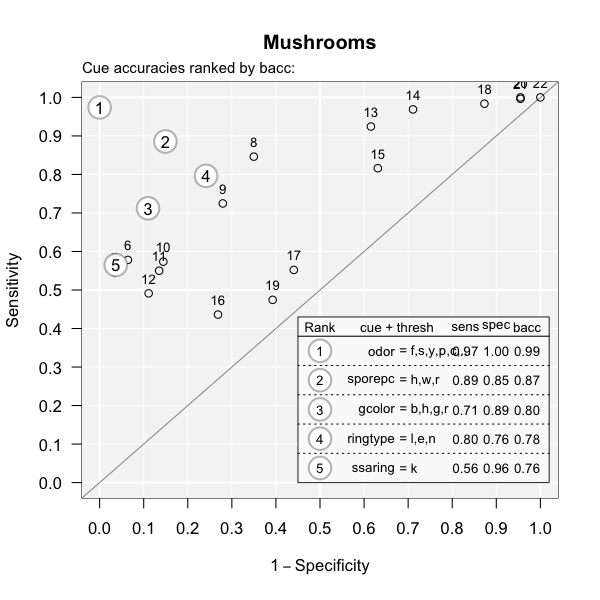It looks like the cues oder and sporepc are the best predictors. In fact, the single cue odor has a hit rate of $$97$$% and a false alarm rate of nearly $$0$$%! Based on this, we should expect the final trees to use just these cues.

#### Visualizing FFT performance

Now let’s plot the performance of the best training tree when applied to the test data:

# Plot the best FFT (for test data):
plot(mushrooms_fft, data = "test")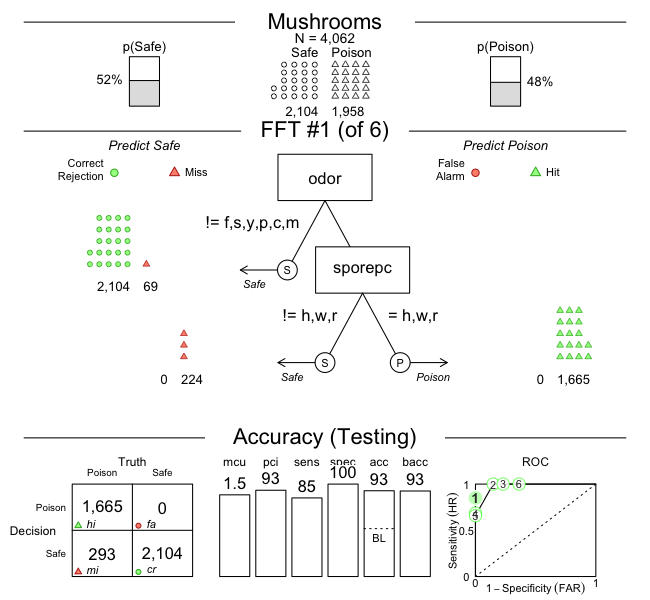Indeed, it looks like the best tree only uses the odor and sporepc cues. In our test dataset, the tree had a false alarm rate of $$0$$% ($$1 -$$ specificity), and a sensitivity (aka. hit rate) of $$85$$%.

When considering the implications of our predictions, the fact that our FFT incurs many misses, but no false alarms, is problematic: Given our current task, failing to detect poisonous mushrooms has usually more serious consequences than falsely classifying some as poisonous. To change the balance between both possible errors, we can select another tree from the set of FFTs. In this case, FFT #2 would use the same two cues, but alter their exit structure so that our prediction incurs false alarms, but no misses. Alternatively, we could re-generate a new set of FFTs with a higher sensitivity weight value (e.g., increase the default value of sens.w = .50 to sens.w = .67) and optimize the FFTs’ weighted accuracy wacc.

#### An alternative FFT

Let’s assume that a famous mushroom expert insists that our FFT is using the wrong cues. According to her, the best predictors for poisonous mushrooms are ringtype and ringnum. To test this, we build a set of FFTs from only these cues and check how they perform relative to our initial tree:

# Create trees using only the ringtype and ringnum cues:
mushrooms_ring_fft <- FFTrees(formula = poisonous ~ ringtype + ringnum,
data = mushrooms,
train.p = .50,
main = "Mushrooms (ring cues)",
decision.labels = c("Safe", "Poison"),
do.comp = FALSE)

Again, we plot the best training tree, when predicting the cases in the test dataset:

# Plotting the best training FFT (for test data):
plot(mushrooms_ring_fft, data = "test")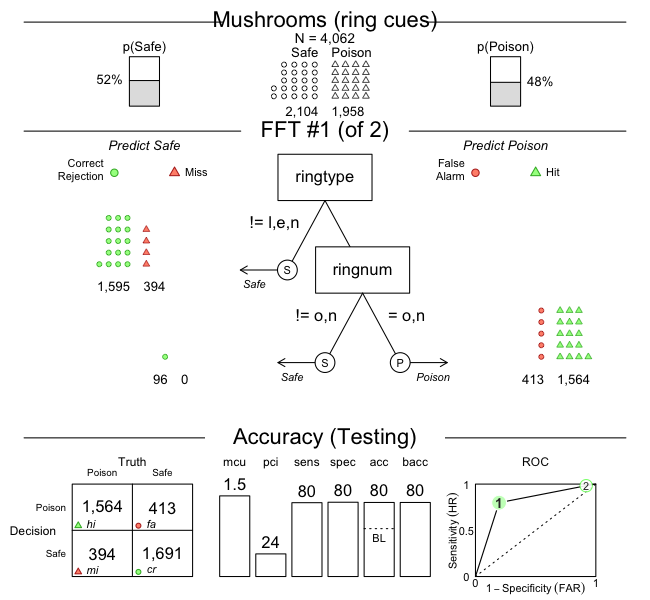As we can see, this tree (in mushrooms_ring_fft) has both sensitivity and specificity values of around $$80$$%, but does not perform as well as our earlier one (in mushrooms_fft). This suggests that we should discard the expert’s advice and primarily rely on the odor and sporepc cues.

### Iris.v dataThe iris.v dataset contains data about 150 flowers (see ?iris.v). Our goal is to predict which flowers are of the class Virginica. In this example, we’ll create trees using the entire dataset (without splitting the available data into explicit training vs. test subsets), so that we are really fitting the data, rather than engaging in genuine prediction:

# Create FFTrees object for iris data:
iris_fft <- FFTrees(formula = virginica ~.,
data = iris.v,
main = "Iris",
decision.labels = c("Not-Vir", "Vir"))

The FFTrees package provides various functions to inspect the FFTrees object iris_fft. For summary information on the best training tree, we can print the FTrees object (by evaluating iris_fft or print(iris_fft)). Alternatively, we could visualize the tree (via plot(iris_fft)) or summarize the FFTrees object (via summary(iris_fft)):

# Inspect resulting FFTs:
print(iris_fft)    # summarize best training tree
plot(iris_fft)     # visualize best training tree
summary(iris_fft)  # summarize FFTrees object

However, let’s first take a look at the individual training cue accuracies…

#### Visualizing cue accuracies

We can plot the training cue accuracies during training by specifying what = "cues":

# Plot cue values:
plot(iris_fft, what = "cues")
#> Plotting cue training statistics:
#> — Cue accuracies ranked by bacc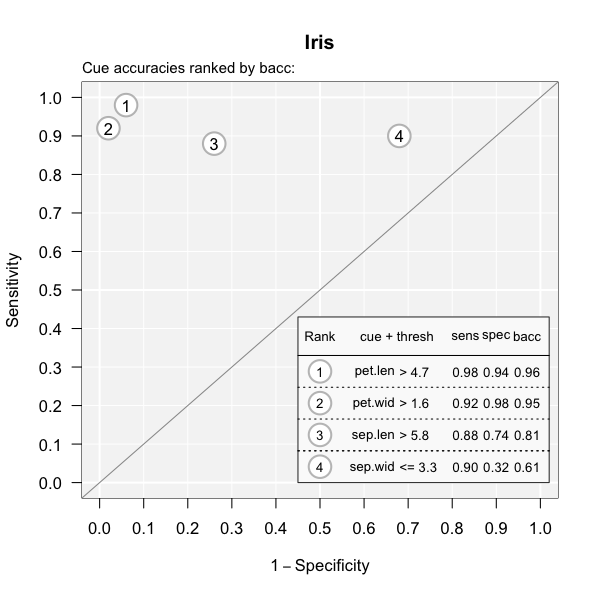It looks like the two cues pet.len and pet.wid are the best predictors for this dataset. Based on this insight, we should expect the final trees will likely use one or both of these cues.

#### Visualizing FFT performance

Now let’s visualize the best tree:

# Plot best FFT:
plot(iris_fft)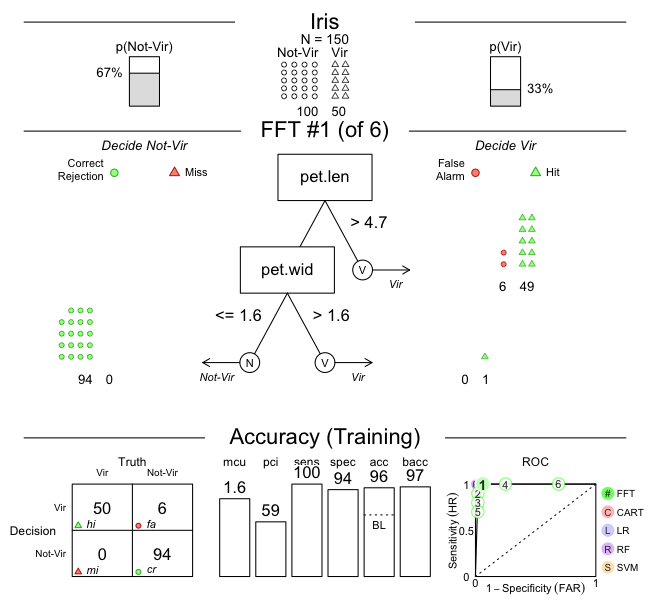Indeed, it turns out that the best tree only uses the pet.len and pet.wid cues (in that order). For this data, the fitted tree exhibits a performance with a sensitivity of 100% and a specificity of 94%.

#### Viewing alternative FFTs

Now, this tree did quite well, but what if someone wanted a tree with the lowest possible false alarm rate? If we inspect the ROC plot in the bottom right corner of the figure, we see that Tree #2 has a specificity close to 100%. Let’s plot this tree:

# Plot FFT #2:
plot(iris_fft, tree = 2)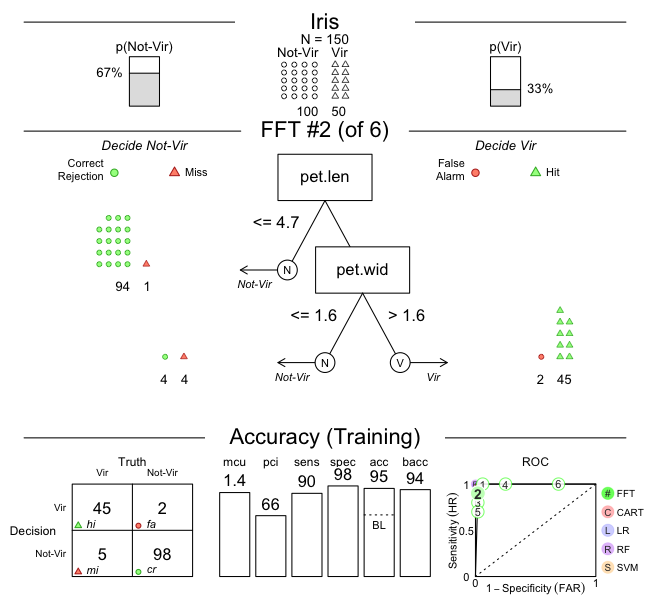As we can see, this tree does indeed have a higher specificity (of 98%), but this increase comes at a cost of a lower sensitivity (of 90%). Such trade-offs between conflicting measures are inevitable when fitting and predicting real-world data. Importantly, using FFTs and the FFTrees package help us to render such trade-offs more transparent.

### Titanic data

For examples that predict people’s survival of the Titanic disaster (by growing FFTs for the titanic data), see the Visualizing FFTs vignette.

## Vignettes

Here is a complete list of the vignettes available in the FFTrees package:

Vignette Description
Main guide: FFTrees overview An overview of the FFTrees package
1 Tutorial: FFTs for heart disease An example of using FFTrees() to model heart disease diagnosis
2 Accuracy statistics Definitions of accuracy statistics used throughout the package
3 Creating FFTs with FFTrees() Details on the main FFTrees() function
4 Manually specifying FFTs How to directly create FFTs without using the built-in algorithms
5 Visualizing FFTs Plotting FFTrees objects, from full trees to icon arrays
6 Examples of FFTs Examples of FFTs from different datasets contained in the package